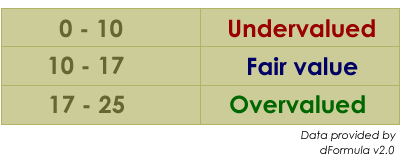# How to Calculate P/E Ratio?

P/E ratio is a good way for investor to calculate how expensive or how cheap a stock is relative to its earnings. In the other words, you can get a sense of whether a stock is overvalued or undervalued.

The ratio is calculated as follows:

P/E = Market Price per share / Earnings per share (EPS)

The earnings per share (EPS) can be obtained in the company financial statement. The lower the P/E, the cheaper the stock is and vice versa. However, high P/E ratio can also be a good thing or a bad thing. It depends on how you look at from different perspective. The low ratio also means that the company stock is currently undervalued, so if you can invest during this period then you can make some handsome profit when the share price go up later.

For example, if the company’s share price is RM1 and the EPS is only RM0.10, then the P/E ratio would be 1/0.1 = 10. If looking at the P/E ratio point of view, the higher the P/E ratio, the more overvalued the company is. Thus, do you think is it risky to buy that share? In this scenario, you should always follow the guideline to determine whether it’s overvalued or undervalued. Refer the guideline as follows: